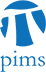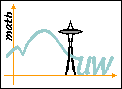## UW-PIMS Colloquia

• Date: 04/30/2010
Lecturer(s):
Ravi Vakil (Stanford University)
Location:

University of Washington

Topic:

Generalizing the Cross Ratio: The Moduli Space of n Points on the Projective Line Up to Projective Equivalence

Description:

Four ordered points on the projective line, up to projective equivalence, are classified by the cross ratio, a notion introduced by Cayley. This theory can be extended to more points, leading to one of the first important examples of an invariant theory problem, studied by Kempe, Hilbert, and others. Instead of the cross ratio (a point on the projective line), we get a point in a larger projective space, and the equations necessarily satisfied by such points exhibit classical combinatorial and geometric structure. For example, the case of six points is intimately connected to the outer automorphism of S6. We extend this picture to an arbitrary number of points, completely describing the equations of the moduli space. This is joint work with Ben Howard, John Millson, and Andrew Snowden. This talk is intended for a general mathematical audience, and much of the talk will be spent discussing the problem, and an elementary graphical means of understanding it.

Schedule:

2:30pm, Room 045, Electrical Engineering Building.